# Functions in Javascript

Javascript Functions

Well, many a time, functions are of great help to us. It is just a block of code that executes whenever it is called. You can say that a function is a block of code that does something in our program (performs some task in our program). You can write different functions for doing different things in JavaScript you can make a function that calculates the sum of numbers for you, a function that greets you, or a function that does something else.

So, here we will be learning how can we create functions in JavaScript, and let me tell you that it is very easy.

## Functions in Javascript

But why should I write functions?

Well, you write the function for once and can use it whenever you want to, in the program. So, isn’t it great to create functions so that we can make that block of code run for us whenever we call it?

Now, after we know a little about functions, you might be wondering that… HOW CAN I CREATE FUNCTIONS??
it is easy… You just have to use the keyword function followed by a function name(You should give a name to a function since you are going to call it). We have to do some things like a function declaration, and function calling as well(as you read above, the function executes when it is called).

Well, first of all, what we are going to do now, is referred to as function declaration(or function definition). Here, we are going to write the keyword function, and then we are going to write the following things –

• as said earlier, we are going to give a name to the function.
• A comma-separated list of required parameters for the function is enclosed in parentheses. (this is written only if required, else the parentheses are empty, which means that no parameters are passed, as shown in the below example)
• the code that you want to put into the function, enclosed in curly brackets.

You can refer to the below program to understand the usage of functions.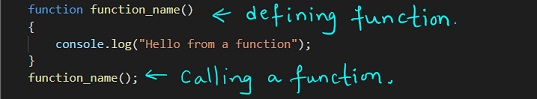Have a look at the output of the very tiny program that we have performed above. The function does nothing, but output as ‘Hello from a function’ to the console.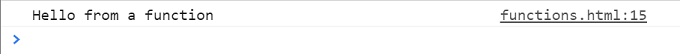Also, now let’s consider an example, through which, we can get an idea of adding parameters to the function. Have a look at the below program here.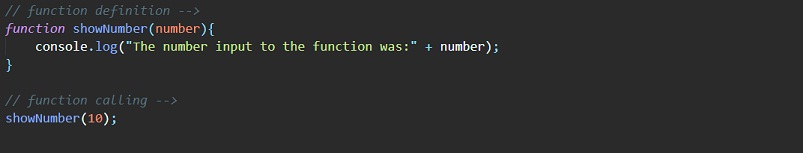Here, in the above example, you can see that there is a function that shows a number, which accepts one parameter called a number. Inside the function, we are just getting the data to the console here. So, whenever we call the show number function, we are going to provide some value to it, and then it will do things accordingly. As you can see in the above example, we are calling the function as well.

As a result, we get something like this on the console –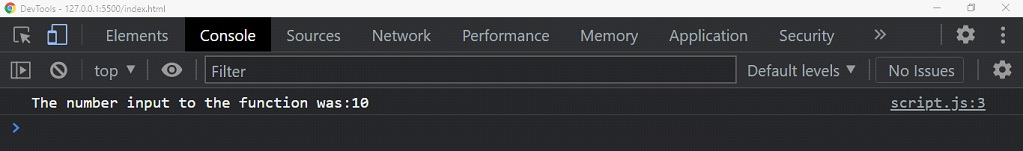As you can see, we got the desired output here. Also, the thing is that we could use the data that we had passed to the function, inside the function.

You can say for understanding, that we are calling the function by its name, like – ” Hey show number, take this number, and run your code”.

We can give multiple parameters to the function as well. We have to provide just the comma-separated list of parameters.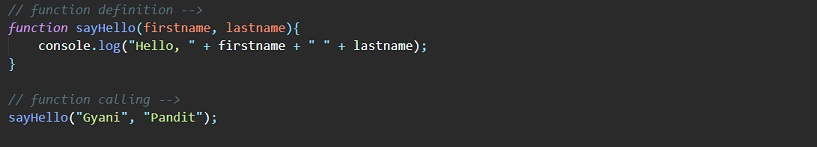If we try to have a look into the console, the output is something like this –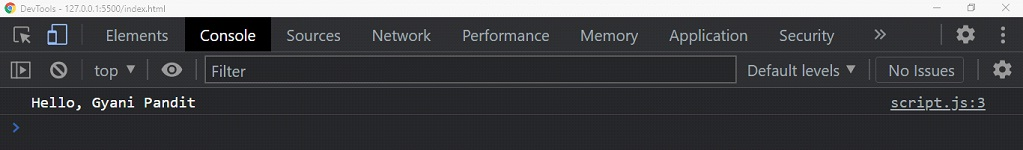So here, we could give multiple values to the function here, and use them in the function. Also, one more thing we can do is to give some default values to the parameter. This is pretty useful when the function has not got the parameter, but it requires it, then it will just have the default value. Have a look here at the program, through which, we can understand the same thing –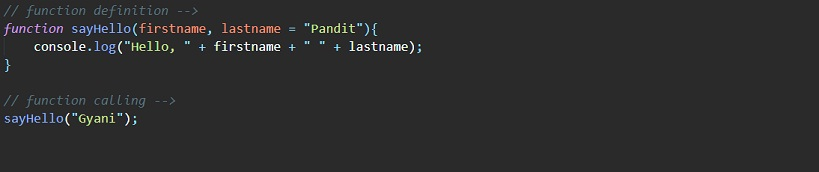As you can see, we have given a default value to the parameter the last name. Also, we forgot to give the last name value, which was a required parameter to the function, but since the parameter has got a default value, it will take that.

Also, another thing is that we can call functions as many times as we want. We have to just write them once, and then use them anytime in the program. We just need to call them. Have a look at the below example, where we are calling some functions multiple times.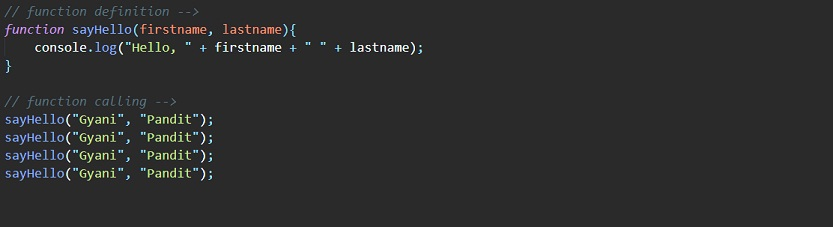As you can see, we could call the function multiple times. If we try to open the console now to have a look at the output, the output comes out to be something like this –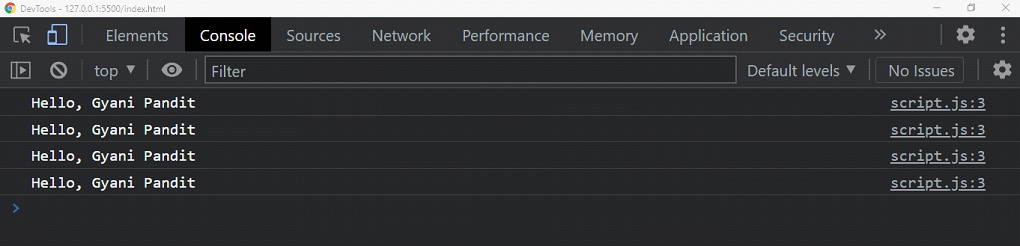As you can find one obvious thing from the above-discussed examples, defining a function is not executing it. Defining is just a specification of what the function is going to do when called. When we are calling the function, the specified actions are being performed, as you can see from several examples taken previously.

We can also return some value from the function. Let’s consider that we have created a function, which accepts a parameter(let’s get it as a number), and then multiplies it with 2, and then returns the new value. This is like… ” hey function… take this number… multiply it with 2, and give it back to me!!”.

Let’s have a look at how this happens. We are going to make use of the return keyword to return something.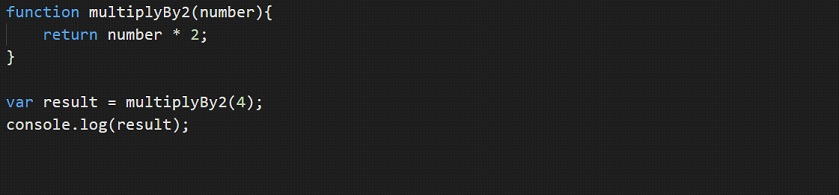As you can see, we are calling the multiplyBy2 function, and then it is multiplying the number(which was passed as a parameter) by 2, and then returning the result. The returned value is stored in another variable, which we have created with the name result, and lastly, we are getting the result on the console. Here is the output for the above program.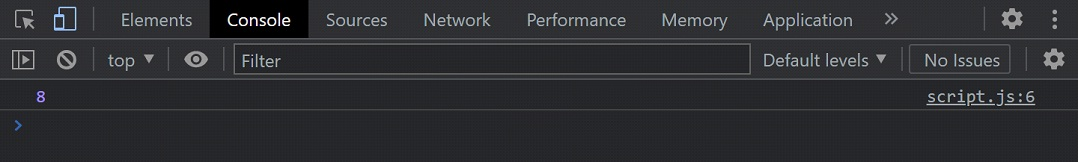As you can see, the function returned the value, which we could use later in the program. This is pretty interesting and useful.